#### Groups

The equivalence relation induced by homotopy starts to enter the realm of algebraic topology, which is a branch of mathematics that characterizes the structure of topological spaces in terms of algebraic objects, such as groups. These resulting groups have important implications for motion planning. Therefore, we give a brief overview. First, the notion of a group must be precisely defined. A group is a set,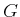, together with a binary operation,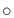, such that the following group axioms are satisfied:

1. (Closure) For any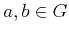, the product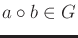.
2. (Associativity) For all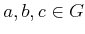,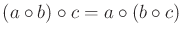. Hence, parentheses are not needed, and the product may be written as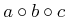.
3. (Identity) There is an element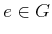, called the identity, such that for all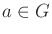,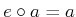and.
4. (Inverse) For every element, there is an element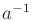, called the inverse of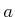, for which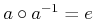and.
Here are some examples.

Example 4..7 (Simple Examples of Groups)   The set of integers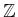is a group with respect to addition. The identity is 0, and the inverse of eachis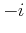. The set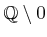of rational numbers with 0 removed is a group with respect to multiplication. The identity is, and the inverse of every element,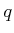, is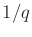(0 was removed to avoid division by zero).An important property, which only some groups possess, is commutativity: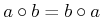for any. The group in this case is called commutative or Abelian. We will encounter examples of both kinds of groups, both commutative and noncommutative. An example of a commutative group is vector addition over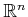. The set of all 3D rotations is an example of a noncommutative group.

Steven M LaValle 2020-08-14